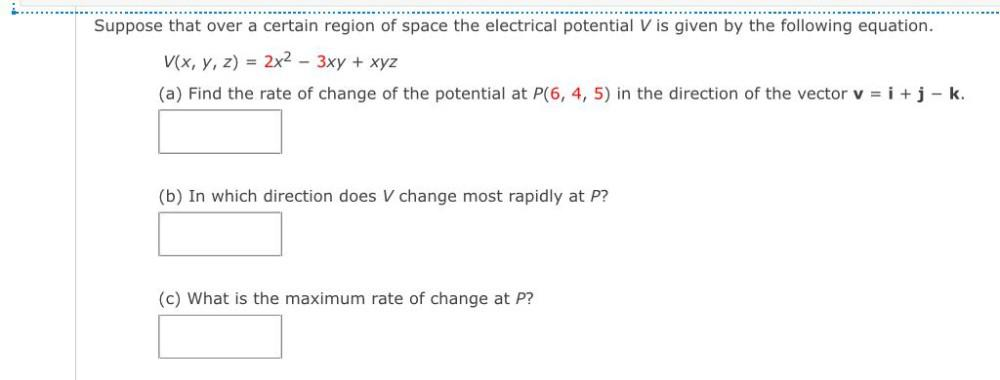Question:

# Suppose that over a certain region of space the electrical potential V is given by the following equation. V(x, y, z) = 2x2 – 3xSuppose that over a certain region of space the electrical potential V is given by the following equation. V(x, y, z) = 2x2 – 3xy + xyz (a) Find the rate of change of the potential at P(6, 4, 5) in the direction of the vector v = i + j-k. (b) In which direction does V change most rapidly at P? (c) What is the maximum rate of change at P?